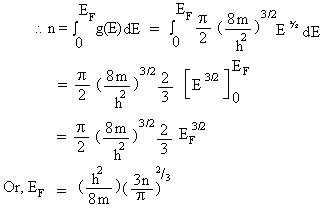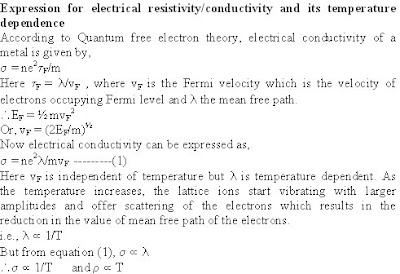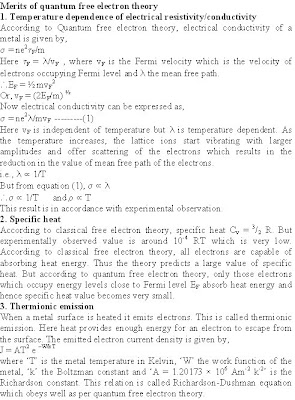Custom Search

## Friday, July 25, 2008

### CLASSICAL FREE ELECTRON THEORY OF METALS

VTU Model Question paper
3.a.I In a metal, if the temperature is increased, then resistivity
A) Increases B) decreases C) remains same D) none of these
II The T > 0K probability of occupancy of Fermi level is
A) 75% B) 90% C) 100% D) 50%
III The electron mobility in metals is given by
A) vd/V B) vd/E C) vd/L D) vd/t
IV The Fermi factor for E = EF at any temperature is
A) 1 B) ½ C) 0 D) 2
b. Define drift velocity, mobility and relaxation time for free electron, derive the expression for conductivity in metals.
c. The Fermi level in Silver is 5.5 eV. What is the energies for which the probabilities of occupancy at 300 K are 0.99 and 0.01. (4+10+6)
December 08 / January 09
3 a. 1) If the mobility of electron in a metal increases, the resistivity
i) Decreases ii) Increases iii) Remains constant iv) none of these.
2) Ohms law relates to the electric field E, conductivity s and current density J as
i) J = E/s ii) J = sE2 iii) J = s/E iv) J = sE
3) The average drift velocity vd of electrons in a metal is related to the electric field and collision time t as
4) Experimentally specific heat at constant volume Cv is given by
i) 3/2 R ii) 10 -4 RT iii) 2/3 R iv) 10 -4 R (04 Marks)
b.Write down the assumptions of classical free electron theory. (04 Marks)
c.Explain failure of classical free electron theory (06 Marks)
d.Find the temperature at which there is 1% probability that a state with an energy 0.5 eV above Fermi energy is occupied. (06 Marks)
June-July 2009
3 a. i) If the mobility of the electron is 7 × 10-3 m2/vs, when accelerated by a field 1v/cm, the vd is given by
A) 7 × 10-3 m/s B) 0.7 m/s C) 7 × 10 -2 m/s D) 0.007 m/s
ii) The temperature dependence for electrical resistivity of metal is
A) r µ 1/T B) r µ 1/ÖT C) rµÖT D) rµ T
iii) The fermi factor for E = EF at T > 0K is
A) 1 B) ½ C) 0 D) 2
iv)According to quantum free electron theory, the energy level in a metal are
A) Continuous B) Discrete C) Overlapping D) None (04 Marks)
b.Derive an expression for density of states for conduction electron for unit volume of metal. (08 Marks)
c.Discuss the various drawbacks of classical free electron theory of metals. What are the assumptions made in quantum theory to overcome (08 Marks)
December 09 / January 10
3 a. i) For ordinary metals, the resistivity versus temperature curve at T = 0,
A) has a positive intercept B) has a negative intercept
C) goes through the origin D) none of these
ii) Which one of the following is correct for current density
A) J = neAvd B) J = neA/vd C) J = nevd D) J = 1/nevd
iii) The value of Fermi distribution function at absolute zero (T= 0 K) is 1, under the condition
A) E = EF B) E > EF C) E >> EF D) E < EF
iv) If the Fermi energy of silver is 5.5 eV, the Fermi velocity of conduction electron is
A) 0.98 × 106 m/s B) 1.39 × 106 m/s C) 2.46 × 105 m/s D) None of these (04 Marks)
b. Describe how quantum free electron theory has been successful in overcoming the failures of classical free electron theory. (06 Marks)
c. What is Fermi energy? Discuss variation of Fermi factor with energy and temperature. (06 Marks)
d. Calculate the probability of an electron occupying an energy level 0.02 eV above the Fermi level at 200 K, in a material. (04 Marks)
May/June 2010
3 a i) The collision time and root mean square velocity of an electron at room temperature are 3×10-14 sec and 1×105 m/s respectively. The classical value of mean free path of the electron is
A) 3×10-19 nm B) 3Å C) 3 nm D) 17.3 nm
ii) Mobility of electron is
A) Reciprocal of conductivity B) Flow of electrons per unit
C) Reciprocal of resistivity D) Average electron drift velocity per unit electric field
iii) The quantum mechanical expression for electrical conductivity is
A) s = m*vF/ne2lF B) s = ne2lF/m2vF C) s = m*vF/n2e2lF D) s = m*/ne2lF
iv) If the Fermi energy of metal at 00 K is 5 eV, f(E) for Fermi energy at T>00K is
A) 0.5 eV B) 1 eV C) 0.75 eV D) 0 eV (04 Marks)
b. Define relaxation time and discuss the dependence of electrical resistivity of metals with temperature and impurity. (06 Marks)
c. Explain how quantum free electron theory succeeds in overcoming the drawbacks of classical free electron theory. (06 marks)
d. A uniform silver wire has resistivity 1.54×10-8 ohm-m at room temperature for an electric field 2 v/m. Calculate relaxation time and drift velocity of the electrons, assuming that there are 5.8×1022 conduction electrons per cm3 of the material. (04 marks)
January 2011
i) The mobility of electrons in a conductor is 4×10-3 m2V-1s-1. Then the drift velocity of the electron in the presence of applied electric field of strength 100 Vm-1 is
A) 4 ms-1 B) 10 ms-1 C) 0.4 ms-1 D) 0.04 ms-1
ii) The classical value of molar specific heat of a conductor is
A) 3/2 R B) ½ R C) 3R D) 5/2 R
iii) The Fermi energy of a metal at absolute zero temperature is proportional to
A) n1/3 B) n3/2 C) n2/3 D) n2
iv) At 50K, the probability of finding an electron at Fermi energy is ½. The probability of finding electron at the same energy level at 100 K is
A) 1 B) zero C) ¼ D) ½ (04 Marks)
b. Obtain the expression for electrical conductivity on the basis of free electron theory of metals. (08 Marks)
c. Explain Fermi energy and Fermi factor. (04 Marks)
d. Calculate the probability of an electron occupying an energy level 0.02 eV above the Fermi level and 0.02 eV below the Fermi level at 200 K. (04 Marks)

December 2011
i) For ordinary metals, resistivity versus temperature curve at T = 0K
A) has a positive intercept   B) has a negative intercept   C) goes through the origin   D) none of these
ii)At T> 0K, probability of occupancy of Fermi level is
A) 75%       B) 90%   C) 100%    D) 50%
iii) If the mobility of electron in a metal increases, the resistivity
A) decreases    B)increases    C) remains constant    D) none of these
iv) The dependence of mean free path λ on temperature T is
A) λ µ T   B) λ µ ÖT   C) λ µ 1/ T  D) λ µ 1/ÖT      (04 Marks)
b. Using the free electron theory, derive an expression for electrical conductivity in metals. (05 Marks)
c. Explain Fermi energy and Fermi factor. (06 Marks)
d. Calculate the Fermi velocity and mean free path for the conduction electrons in silve, given that its Fermi energy is 5.5eV and the relaxation time for electrons is 3.97×10-14 s.    (05 Marks)
June 2012
i) In classical free electron theory, the electric field due to ion cores
A) is neglected    B) is assumed to be periodic   C) is assumed to be a constant    D) None of these
ii) Mobility of electron is
A) reciprocal of electrical conductivity   B) acceleration of electron per unit electric field   C) average drift velocity per unit electric field   D) None of these
iii) If EF is the Fermi energy at absolute zero, then mean energy of the electron at absolute zero is
A) Ē = 1.5 EF   B) Ē = 2/3 EF   C) Ē = 2/5 EF   D) Ē = 3/5 EF
iv) The resistivity of metals is due to scattering of electron by
A) phonons    B) lattice imperfection    C) impurities    D) All of these    (04 Marks)
b. Explain the terms
i) Relaxation time ii) Mean collision time   iii) Drift velocity   (06 Marks)
c. Define Fermi energy. Discuss the Fermi factor f(σ) for cases EF
, E>EF at T=0, E=EF at T≠0.       (05 Marks)
d. Calculate the conductivity of sodium. Given τm = 2×10-14 s. Density of sodium is 971 kg/m3. Its atomic weight is 23 and has one conduction electron/atom.   (05 Marks)
Free electron conceptAll metal atoms consist of valence electrons which are responsible for electrical conduction. Valence electrons are loosely bound to the nucleus. When a large number of atoms join to form a metal, the boundaries of the neighbouring atoms overlap, and thus, valence electrons can move easily throughout the body of the metal. These electrons are called free electrons or conduction electrons which account for properties such as electrical conductivity, thermal conductivity, opacity, surface luster etc.
Classical free electron theoryThis theory was developed by Drude and Lorentz and hence is also known as Drude-Lorentz theory. According to this theory, a metal consists of electrons which are free to move about in the body of the metal like molecules of a gas in a container.
Assumptions1. A metal is imagined as a structure of 3-dimensional array of ions between which, there are freely moving valence electrons (conduction electrons) confined to the body of the material.
2. Mutual repulsion between electrons is ignored and hence potential energy is taken as zero. Therefore the total energy of the electron is equal to its kinetic energy.
3. The free electrons are treated as equivalent to gas molecules and thus they are assumed to obey the laws of kinetic theory of gases. In the absence of field, the energy associated with each electron at a temperature T is given by 3/2 kT.
4. Drift velocityIf no electric field is applied on a conductor, the free electrons move in random directions. They collide with each other and also with the positive ions. Since the motion is completely random, average velocity in any direction is zero. If a constant electric field is established inside a conductor, the electrons experience a force F = -eE due to which they move in the direction opposite to direction of the field. These electrons undergo frequent collisions with positive ions. In each such collision, direction of motion of electrons undergoes random changes. As a result, in addition to the random motion, the electrons are subjected to a very slow directional motion. This motion is called drift and the average velocity of this motion is called drift velocity vd.5. Positive Hall co-efficient of Zinc
Metals are expected to exhibit negative Hall co-efficient since current carriers in them are electrons. The free electron theory cannot explain why Zinc and some other metals have positive Hall co-efficient.
6. The free electron theory cannot explain the classification of materials into conductors, semiconductors and insulators.
7. This theory fails to explain ferromagnetism, superconductivity, photoelectric effect, Compton effect and blackbody radiation.
Quantum free electron theory
Classical free electron theory could not explain many physical properties. In classical free electron theory, we use Maxwell-Boltzman statistics which permits all the free electrons to gain energy. In 1928, Sommerfeld developed a new theory, in which he retained some of the features of classical free electron theory and included quantum mechanical concepts and Fermi-Dirac statistics to the free electrons in the metal. This theory is called quantum free electron theory. Quantum free electron theory permits only a fraction of electrons to gain energy. The main assumptions of this theory are:
1. The energy values of conduction electrons are quantized and are realized in terms of a set of energy levels.
2. The distribution of electrons in various allowed energy levels takes place according to Pauli’s exclusion principle.
3. The electrons move in a constant potential inside the metal and are confined within defined boundaries.
4. The attraction between the electrons and the lattice ions and the repulsion between the electrons themselves are ignored.Classical free electron theory and quantum free electron theory-Comparison
Similarities
1. Valence electrons are treated as gas molecules are treated as gas molecules of an ideal gas which are free to move throughout the body of the solid.
2. The mutual repulsion between the electrons and the force of attraction between the electrons and ions are considered insignificant.
Differences
1. According to classical free electron theory, free electrons obey Maxwell-Boltzman statistics. According to quantum free electron theory, free electrons obey Fermi-Dirac statistics.
2. According to classical free electron theory, free electrons can possess any energy values and it is possible that many electrons possessing same energy. According to quantum free electron theory, free electrons can occupy certain energy levels with discrete energy values and they obey Pauli’s exclusion principle. Hence no two electrons possess same energy.Demerits of quantum free electron theory
1. It fails to explain properties of metal alloys.
2. It fails to explain why only some solids are metals and others are either semiconductors or insulators.